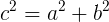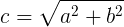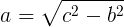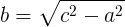# Pythagorean theorem calculator

Hypotenuse (c) calculation:

 Enter leg (a): Enter leg (b): Hypotenuse (c) result:

Leg (a) calculation:

 Enter leg (b): Enter Hypotenuse (c): Leg (a) result:

Leg (b) calculation:

 Enter leg (a): Enter hypotenuse (c): Leg (b) result:

## Pythagorean theorem

For right triangle: the square value the hypotenuse (c) is equal to the sum of the square value of leg (a) and the square value of leg (b):### Hypotenuse (c) calculation### Leg (a) calculation### Leg (b) calculation